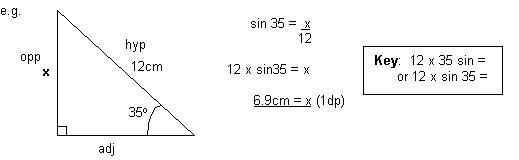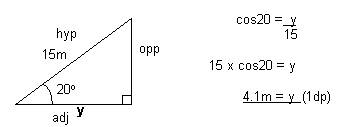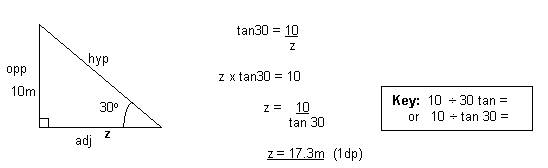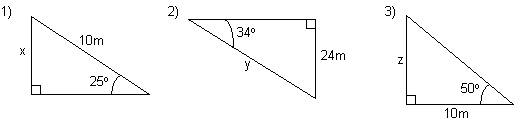# Calculating sides using trigometric ratios - Math Review

math tutorials > calculating sides of a triangle using trig ratios

## Solving trig ratios - what you will need:

To solve trigonometric ratios you will need a scientific calculator.  Depending on whether you have a Direct Algebra Logic (DAL) calculator or not, the order you key in the calculation may vary.  Experiment until you get the results shown here.

## Steps

1. Label the sides (o, a, h)
2. Identify which trig. ratio uses these sides.
3. Calculate the side.## Trigonometry problems for you to try.Solutions:  1)  4.2m  (1dp)     2)  28.9m  (1dp)     3)  8.4m  (1dp)